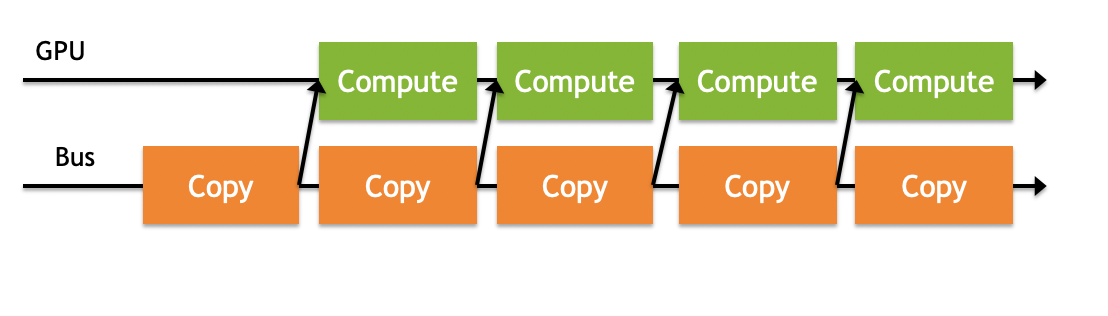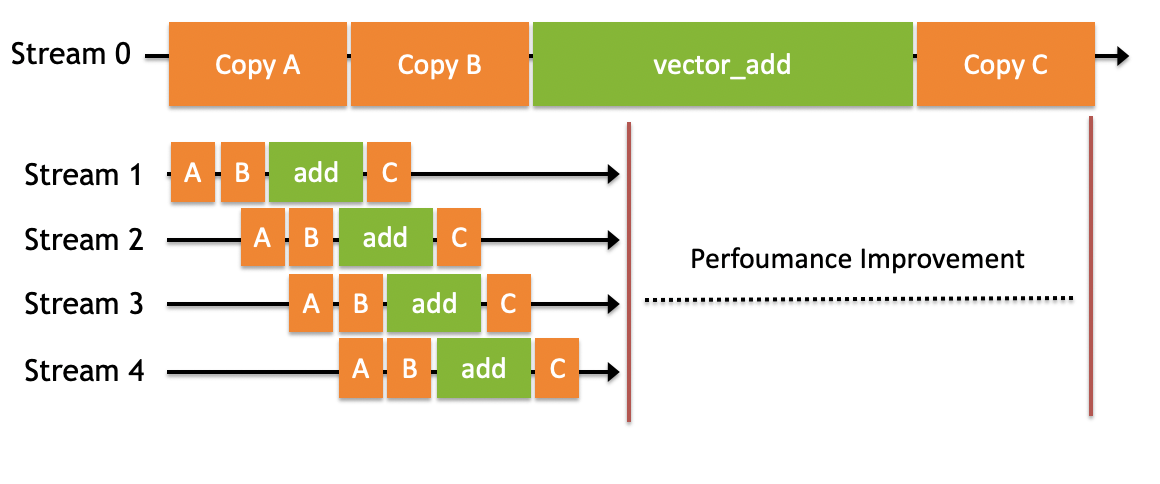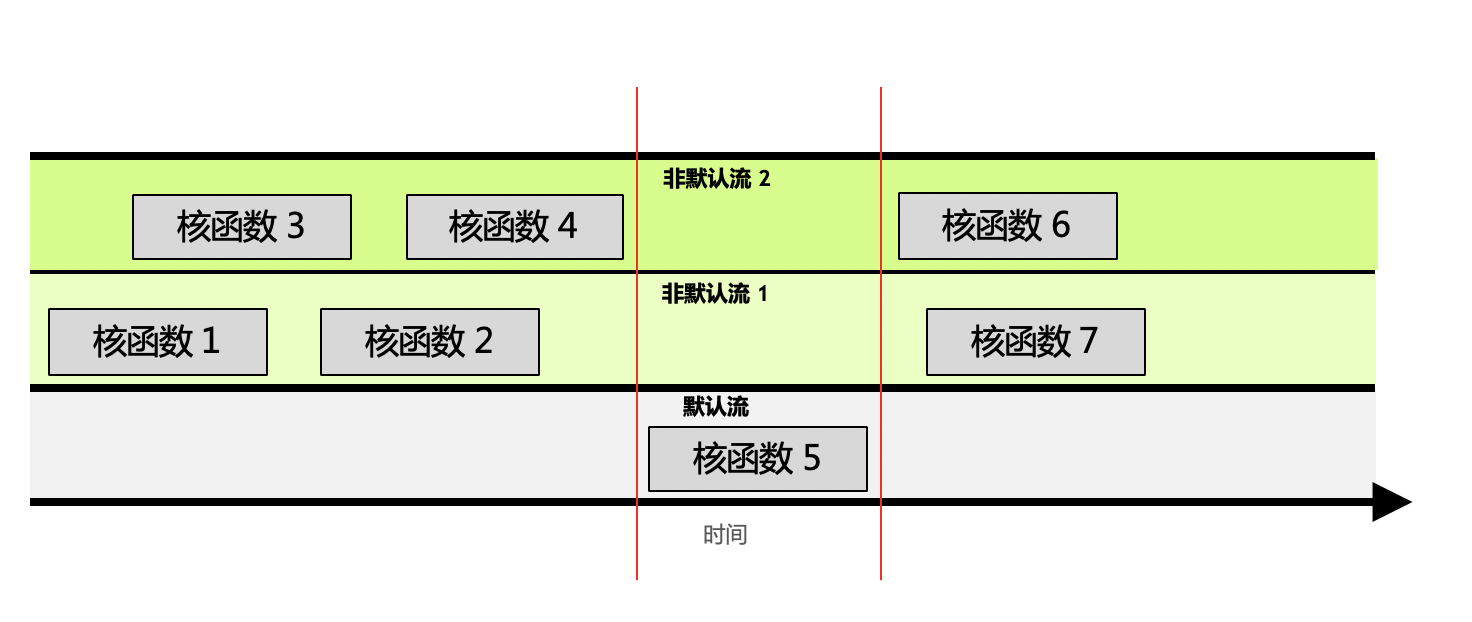# 多流

• 主机端上的计算
• 设备端的计算（核函数）
• 数据从主机和设备间相互拷贝
• 数据从设备内拷贝或转移
• 数据从多个GPU设备间拷贝或转移• 给定流内的所有操作会按序执行。
• 非默认流之间的不同操作，无法保证其执行顺序。
• 所有非默认流执行完后，才能执行默认流；默认流执行完后，才能执行其他非默认流。• 非默认流1中，根据进流的先后顺序，核函数1和2是顺序执行的。
• 无法保证核函数2与核函数4的执行先后顺序，因为他们在不同的流中。他们执行的开始时间依赖于该流中前一个操作结束时间，例如核函数2的开始依赖于核函数1的结束，与核函数3、4完全不相关。
• 默认流有阻塞的作用。如图中红线所示，如果调用默认流，那么默认流会等非默认流都执行完才能执行；同样，默认流执行完，才能再次执行其他非默认流。

stream = numba.cuda.stream()

CUDA的数据拷贝以及核函数都有专门的stream参数来接收流，以告知该操作放入哪个流中执行：

• numba.cuda.to_device(obj, stream=0, copy=True, to=None)
• numba.cuda.copy_to_host(self, ary=None, stream=0)

• kernel[blocks_per_grid, threads_per_block, stream=0]

from numba import cudaimport numpy as npimport mathfrom time import time
@cuda.jitdef vector_add(a, b, result, n):    idx = cuda.threadIdx.x + cuda.blockDim.x * cuda.blockIdx.x    if idx < n :        result[idx] = a[idx] + b[idx]
def main():    n = 20000000    x = np.random.uniform(10,20,n)    y = np.random.uniform(10,20,n)    # x = np.arange(n).astype(np.int32)    # y = 2 * x
start = time()    # 使用默认流    # Host To Device    x_device = cuda.to_device(x)    y_device = cuda.to_device(y)    z_device = cuda.device_array(n)    z_streams_device = cuda.device_array(n)
# Kernel    vector_add[blocks_per_grid, threads_per_block](x_device, y_device, z_device, n)        # Device To Host    default_stream_result = z_device.copy_to_host()    cuda.synchronize()    print("gpu vector add time " + str(time() - start))
start = time()
# 使用5个流    number_of_streams = 5    # 每个流处理的数据量为原来的 1/5    # 符号//得到一个整数结果    segment_size = n // number_of_streams
# 创建5个cuda stream    stream_list = list()    for i in range (0, number_of_streams):        stream = cuda.stream()        stream_list.append(stream)
threads_per_block = 1024    # 每个stream的处理的数据变为原来的1/5    blocks_per_grid = math.ceil(segment_size / threads_per_block)    streams_result = np.empty(n)
# 启动多个stream    for i in range(0, number_of_streams):        # 传入不同的参数，让函数在不同的流执行                # Host To Device        x_i_device = cuda.to_device(x[i * segment_size : (i + 1) * segment_size], stream=stream_list[i])        y_i_device = cuda.to_device(y[i * segment_size : (i + 1) * segment_size], stream=stream_list[i])
# Kernel        vector_add[blocks_per_grid, threads_per_block, stream_list[i]](                x_i_device,                y_i_device,                z_streams_device[i * segment_size : (i + 1) * segment_size],                segment_size)
# Device To Host        streams_result[i * segment_size : (i + 1) * segment_size] = z_streams_device[i * segment_size : (i + 1) * segment_size].copy_to_host(stream=stream_list[i])
cuda.synchronize()    print("gpu streams vector add time " + str(time() - start))
if (np.array_equal(default_stream_result, streams_result)):        print("result correct")
if __name__ == "__main__":    main()

gpu vector add time 0.8897650241851807gpu streams vector add time 0.20893597602844238